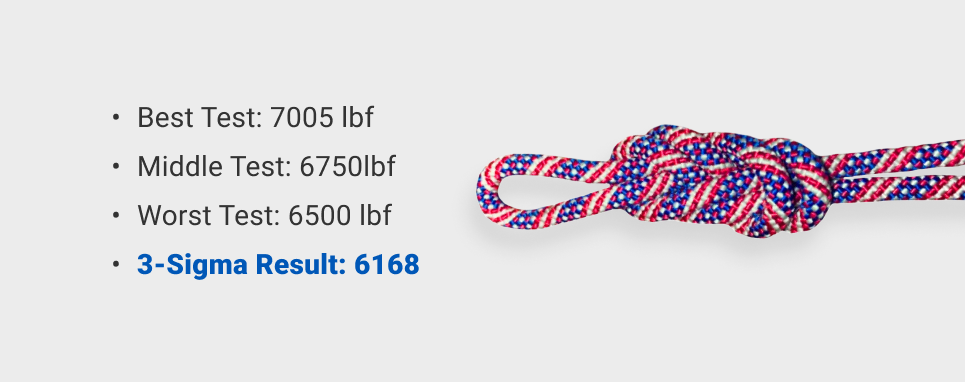### ⊕ USA most respected life safety rope standards (NFPA 2500 and ANSI Z359 and ANSI Z459) refer back to Cordage Institute Life Safety Rope Test Methods (CI1801).

• Unique method of reporting Minimum Breaking Strength.
• break testing at least five samples of rope
• calculating three standard deviations below the mean of those results.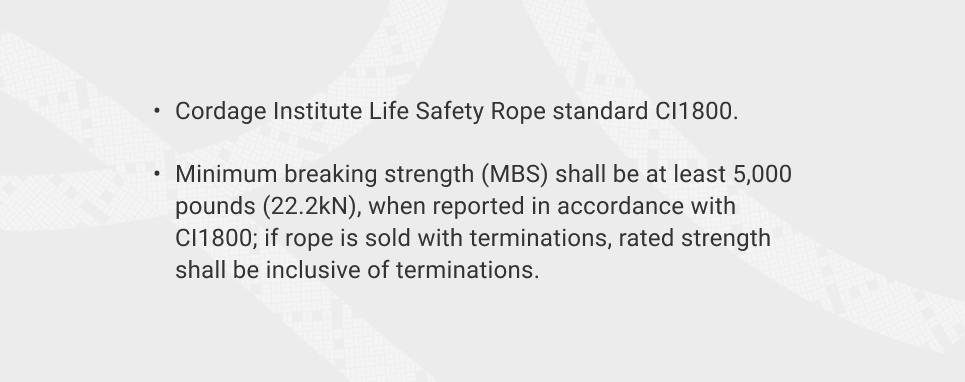### ⊕ EXAMPLE: 5 samples from five different lots of rope

• Lab wll pull test the rope to failure.
• Inevitably slightly different results with each test.
• what is the RIGHT number to report?

### ⊕ Actual numbers Scenario

• (6500) pounds
• (6750) pounds
• (6675) pounds
• (7005) pounds
• (6925) pounds

### ⊕ What number do we report?

• best result?  7005 pounds force.
• worst-case? 6500 pounds (about 500 pounds less than the best result)
• What aboutnatural variation in materials testing – are these reliable?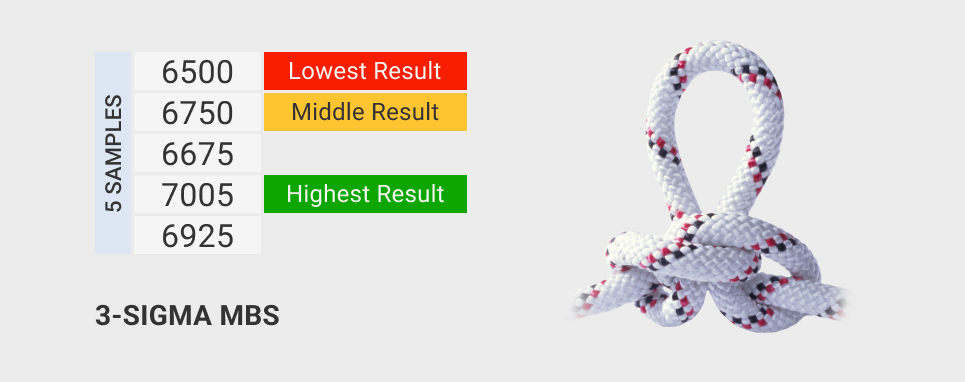### ⊕ Cordage Institute test method (and the NFPA and ANSI standards that refer back to it)

• mathematical approach to ensure statistical significance
• Other Standdards(Euro-norms or CE) do not use this method

### ⊕ At least five tests from different manufacturing lots, very specific test method

• calculate the MEAN AVERAGE
• SUBRACT three standard deviations from the mean
• 7% confidence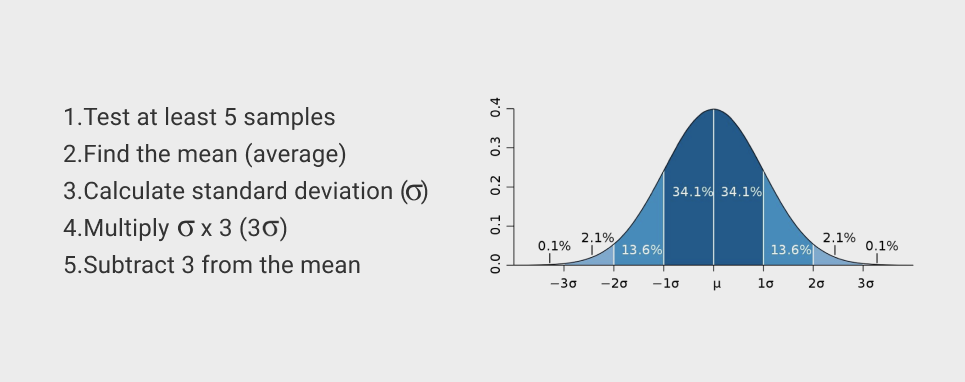### ⊕ Practical view, sample set.

• add up the sum of all our test results (33,855)
• THEN we divide by the number of tests (5)
• Average – 6771
• which is six thousand seven hundred seventy one
• Calculate standard deviation from mean average (200lbf)
• symbol called SIGMA.
• multiply Standard Deviation by THREE…. 3x 200.98 = 602.94
• refer to this as THREE SIGMA
• SUBTRACT that 3-Sigma number from the MEAN AVERAGE
• 6771 – 602.94 = 6168
• 900 pounds less than the best test
• 332 pounds lower than even the worst test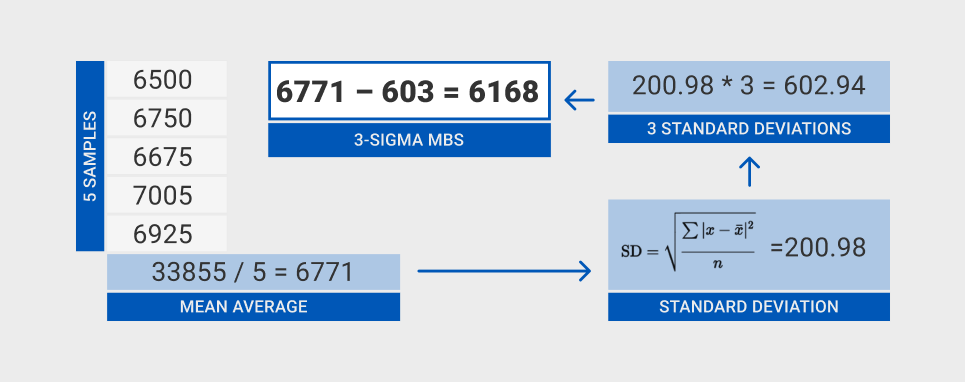### ⊕ THIS WILL affect your safety factor calculations!

• 3s mbs is a number that you can rely on
• 7% confidence

### ⊕ NOT all standards use this method

• even within Cordage Institute, only the Life Safety Rope standard uses it
• many standards don’t even specify how the reported MBS is supposed to be derived
• only that the strength of tested samples needs to be greater than X